Next: Transitions in Hydrogen Up: Time-Dependent Perturbation Theory Previous: Radiation from a Harmonic

# Selection Rules

Let us now consider spontaneous transitions between the different energy levels of a hydrogen atom. Since the perturbing Hamiltonian (1093) does not contain any spin operators, we can neglect electron spin in our analysis. Thus, according to Sect. 9.4, the various energy eigenstates of the hydrogen atom are labeled by the familiar quantum numbers,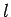, and.

According to Eqs. (1122) and (1131), a hydrogen atom can only make a spontaneous transition from an energy state corresponding to the quantum numbers,,to one corresponding to the quantum numbers,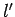,if the modulus squared of the associated electric dipole moment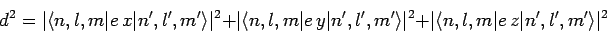(1144)

is non-zero. Now, we have already seen, in Sect. 12.5, that the matrix elementis only non-zero provided thatand. It turns out that the proof that this matrix element is zero unlesscan, via a trivial modification, also be used to demonstrate thatand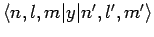are also zero unless. Consider(1145)

It is easily demonstrated that(1146)

Hence,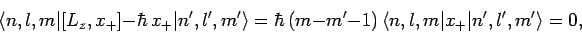(1147)

and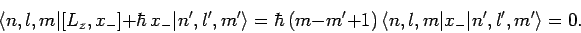(1148)

Clearly,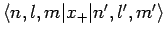is zero unless, andis zero unless. Now,andare obviously both zero ifandare both zero. Hence, we conclude thatandare only non-zero if.

The above arguments demonstrate that spontaneous transitions between different energy levels of a hydrogen atom are only possible provided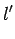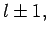(1149)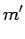(1150)

These are termed the selection rules for electric dipole transitions (i.e., transitions calculated using the electric dipole approximation). Note, finally, that since the perturbing Hamiltonian does not contain any spin operators, the spin quantum number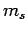cannot change during a transition. Hence, we have the additional selection rule that.Next: Transitions in Hydrogen Up: Time-Dependent Perturbation Theory Previous: Radiation from a Harmonic
Richard Fitzpatrick 2010-07-20